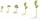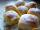# Adding mixed numbers

Add this two mixed numbers: 1 5/6 + 2 2/11=

Result

f =  4.015

#### Solution:Leave us a comment of example and its solution (i.e. if it is still somewhat unclear...):

Showing 0 comments:Be the first to comment!#### To solve this example are needed these knowledge from mathematics:

Need help calculate sum, simplify or multiply fractions? Try our fraction calculator.

## Next similar examples:

1. Addition of mixed numeralsAdd two mixed fractions: 2 4/6 + 1 3/6
2. Roman numerals 2+Add up the number writtens in Roman numerals. Write the results as a roman numbers.
3. This morningThis morning it was 80 degrees F outside the temperature increased 12 degrees F. What is the temperature now?
4. Roses and tulipsAt the florist are 50 tulips and 5 times less roses. How many flowers are in flower shop?
5. Roman numerals +Add up the number writtens in Roman numerals. Write the results as a decimal number.
6. Mixed2improperWrite the mixed number as an improper fraction. 166 2/3
7. Addition of Roman numbersAdded together and write as decimal number: LXVII + MLXIV
8. CakesOn the bowl were a few cakes. Jane ate one-third of them, Dana ate a quarter of those cakes that remained. a) What part (of the original number of cakes) Dana ate? b) At least how many cakes could be (initially) on thebowl?
9. SeedsOf the 72 wet seeds 7/8 germinated. How many seeds germinate?
10. ChocolateChildren break chocolate first to third and then every part of another half. What kind got each child? Draw a picture. What part would have received if each piece have halved?
11. Fraction and a decimalWrite as a fraction and a decimal. One and two plus three and five hundredths
12. Lengths of the poolMiguel swam 6 lengths of the pool. Mat swam 3 times as far as Miguel. Lionel swam 1/3 as far as Miguel. How many lengths did mat swim?
13. Fraction to decimalWrite the fraction 3/22 as a decimal.
14. ZdeněkZdeněk picked up 15 l of water from a 100-liter full-water barrel. Write a fraction of what part of Zdeněk's water he picked.
15. In fractionsAn ant climbs 2/5 of the pole on the first hour and climbs 1/4 of the pole on the next hour. What part of the pole does the ant climb in two hours?
16. Jam cakesMom baked a third of plum jam cakes, one third cheesecakes and 18 poppy. How many cakes she had bake?
17. Cakes1/3 poppy cake, 1/3 apple, 15 pieces of cheese. How many are totally cakes?